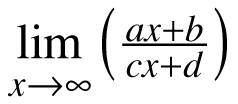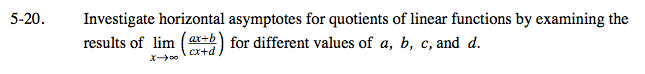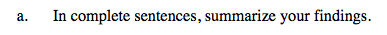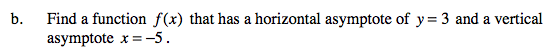### Home > CALC > Chapter 5 > Lesson 5.1.2 > Problem5-20

5-20.
1. Investigate horizontal asymptotes for quotients of linear functions by examining the results offor different values of a, b, c, and d. Homework Help ✎

1. In complete sentences, summarize your findings.

2. Find a function f(x) that has a horizontal asymptote of y = 3 and a vertical asymptote x = −5A horizontal asymptote can be found by evaluating:

(Compare the highest-powered term in the numerator with the highest-powered term in the denominator.)Vertical asymptotes can be found by setting the denominator equal to 0, AFTER you simplify the fraction. As for horizontal asymptotes, see the hint in part (a).

Use the eTool below to visualize the problem.
Click the link at right for the full version of the eTool: Calc 5-20 HW eTool